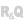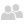HelpWLanguageWLanguage functionsControls, pages and windowsDrawing functionsTypes of variablesPolygonPresentationExampleProperties specific to the description of Polygon variablesWLanguage functions that use the Polygon typeSee alsoBackgroundColorIconImagePalettePolygonTiff
• Properties specific to the description of Polygon variables
• WLanguage functions that use the Polygon type
WINDEVWEBDEVWINDEV MobileOthersPolygon (Type of variable)
In french: Polygone
The Polygon type is used to define the points of a polygon. These points are ordered and connected in the defined order.
The characteristics of this polygon can be defined and changed using different WLanguage properties.
Remark: For more details on the declaration of this type of variable and the use of WLanguage properties, see Declaring a variable.
Example
polTriangle is Polygon
oPoint is Point
oPoint.X = 460
oPoint.Y = 10
oPoint.X = 500
oPoint.Y = 80
oPoint.X = 420
oPoint.Y = 80
dPolygon(polTriangle, PastelBlue, LightGreen)

polRectangle is Polygon
polRectangle.Point = [[420,90], [500,90], [500,150], [420,150]]
dPolygon(polRectangle, DarkBrown)
Remarks

Properties specific to the description of Polygon variables

The following properties can be used to handle a polygon:
Property name Type used Effect Point Array of Point variables Polygon points.

WLanguage functions that use the Polygon type

 dPolygon Draws a polygon: in an Image control, in an Image variable, in a WDPic variable (on the background layer),in a picLayer variable. dPolyline Draws a line composed of multiple segments.
Minimum version required
• Version 26